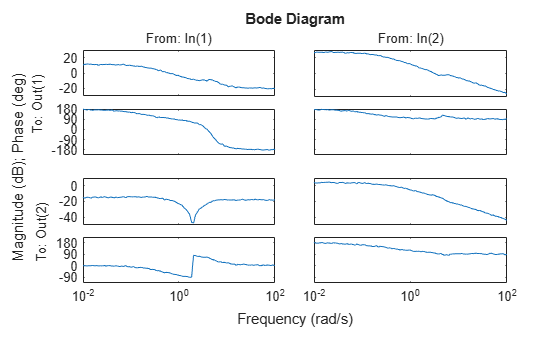# MIMO Frequency Response Data Models

This example shows how to create a MIMO frequency-response model using an `frd` object.

Frequency response data for a MIMO system includes a vector of complex response data for each of the input/output (I/O) pair of the system. Thus, if you measure the frequency response of each I/O pair of your system at a set of test frequencies, you can use the data to create a frequency response model.

For this example, load the frequency response data in `AnalyzerDataMIMO.mat`.

`load AnalyzerDataMIMO H11 H12 H21 H22 freq`

This command loads the data into the MATLAB® workspace as five column vectors `H11`, `H12`, `H21`, `H22`, and `freq`. The vector `freq` contains 100 test frequencies. The other four vectors contain the corresponding complex-valued frequency response of each I/O pair of a two-input, two-output system.

Organize the data into a three-dimensional array.

```Hresp = zeros(2,2,length(freq)); Hresp(1,1,:) = H11; Hresp(1,2,:) = H12; Hresp(2,1,:) = H21; Hresp(2,2,:) = H22;```

The dimensions of `Hresp` are the number of outputs, number of inputs, and the number of frequencies for which there is response data. `Hresp(i,j,:)` contains the frequency response from input `j` to output `i`.

Create a frequency-response model.

`H = frd(Hresp,freq);`

`H` is an `frd` model object that represents frequency response data.

You can use `frd` models with many frequency-domain analysis commands. For example, visualize the response of this two-input, two-output system using `bode`.

`bode(H)`By default, the `frd` command assumes that the frequencies are in radians/second. To specify different frequency units, use the `TimeUnit` and `FrequencyUnit` properties of the `frd` model object. For example, set the frequency units to radians/minute.

`H = frd(Hresp,freq,"TimeUnit","min","FrequencyUnit","rad/TimeUnit");`